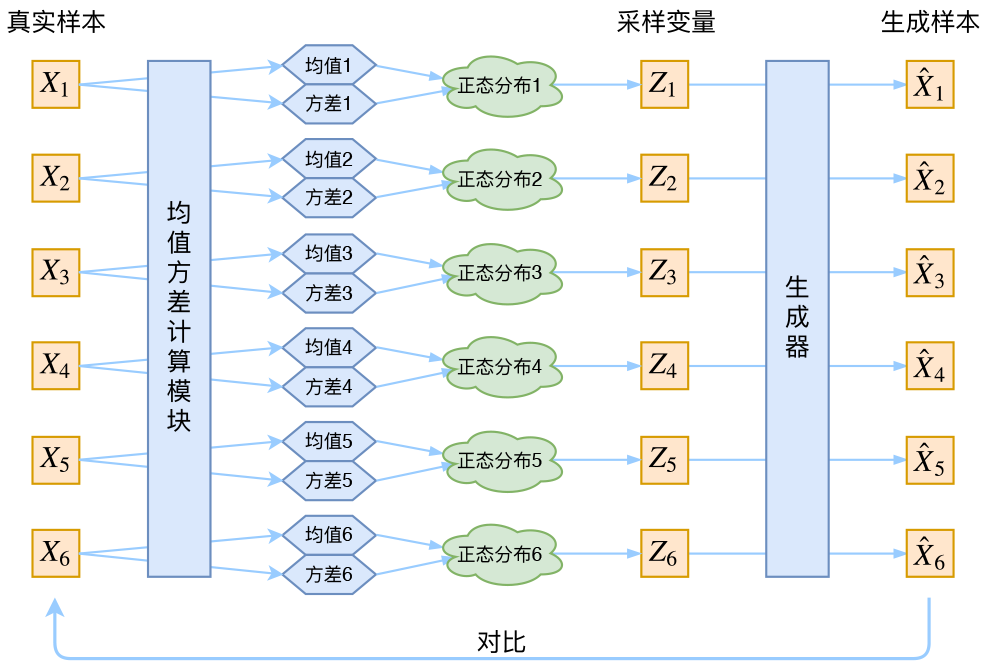# 变分自编码器# 流程

$q(X)=\int q(X,Z) \mathrm{d}Z=\int q(X|Z)q(Z) \mathrm{d}Z$

$p(Z_i)=\int p(Z|x_i)p(X)\mathrm{d}X$

# 推导

## 编码过程

$p(Z)=\int_X p(Z,X)\mathrm{d}X=\int_Xp(Z|X)p(X)\mathrm{d}X=\sum_Xp(Z|X)p(X)$$=\sum_X N(0,1)p(X)=N(0,1)\sum_Xp(X)=N(0,1)$

$KL(p(Z|x_i)||N(0,1))=KL(N(\mu_i,\delta_i)||N(0,1))=\frac12 \sum_i(\mu_i^2+\delta_i^2-\log \delta_i^2-1)$

## 解码过程

$q(X)=\int q(X,Z)\mathrm{d}z=\int q(X|Z)q(Z)\mathrm{d} z$

## Loss

$p(X)=\int p(X,Z)\mathrm{d}z=\int p(X|Z)p(Z)\mathrm{d}z$

$q(X)=\int q(X,Z)\mathrm{d}z=\int q(X|Z)q(Z)\mathrm{d} z$

$KL(p(X,Z)||q(X,Z))=\int p(X)\left [\int p(Z|X) \ln \frac{p(X)p(Z|X)}{q(X,Z)} \mathrm{d} z\right] \mathrm{d} x$
$=\mathbb{E}_{x \sim p(X)} \left [ \mathbb{E}_{x \sim p(Z|X)} [- \ln q(X|Z)] +KL(p(Z|X)||q(Z)) \right]$

# 释疑

## 为啥是p和q

vae分为两个过程，编码过程和解码过程

## p(Z)和q(Z)